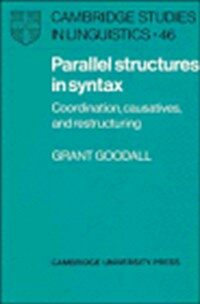> 상세정보

# 상세정보## Parallel structures in syntax : coordination, causatives, and restructuring (2회 대출)

자료유형
단행본
개인저자
Goodall, Grant.
서명 / 저자사항
Parallel structures in syntax : coordination, causatives, and restructuring / Grant Goodall.
발행사항
Cambridge [Cambridgeshire] ;   New York :   Cambridge University Press ,   1987.
형태사항
xiii, 194 p. : ill. ; 24 cm.
총서사항
Cambridge studies in linguistics ; 46
ISBN
052132307X 9780521323079 (hardback) 9780521109161 (paperback)
서지주기
Includes bibliographical references (p. 186-189)and index.
일반주제명
Causative (Linguistics) Generative grammar. Grammar, Comparative and general -- Coordinate constructions. Grammar, Comparative and general -- Syntax. Parallelism (Linguistics)
 000 01210camuu2200325 a 4500 001 000045590523 005 20100503150041 008 100503s1987 enka b 001 0 eng d 010 ▼a 86020790 020 ▼a 052132307X 020 ▼a 9780521323079 (hardback) 020 ▼a 9780521109161 (paperback) 040 ▼a DLC ▼c DLC ▼d DLC ▼d 211009 050 0 0 ▼a P291 ▼b .G66 1987 082 0 0 ▼a 415 ▼2 22 090 ▼a 415 ▼b G646p 100 1 ▼a Goodall, Grant. 245 1 0 ▼a Parallel structures in syntax : ▼b coordination, causatives, and restructuring / ▼c Grant Goodall. 260 ▼a Cambridge [Cambridgeshire] ; ▼a New York : ▼b Cambridge University Press , ▼c 1987. 300 ▼a xiii, 194 p. : ▼b ill. ; ▼c 24 cm. 490 1 ▼a Cambridge studies in linguistics ; ▼v 46 504 ▼a Includes bibliographical references (p. 186-189)and index. 650 0 ▼a Causative (Linguistics) 650 0 ▼a Generative grammar. 650 0 ▼a Grammar, Comparative and general ▼x Coordinate constructions. 650 0 ▼a Grammar, Comparative and general ▼x Syntax. 650 0 ▼a Parallelism (Linguistics) 830 0 ▼a Cambridge studies in linguistics ; ▼v 46. 945 ▼a KLPA

### 소장정보

No. 소장처 청구기호 등록번호 도서상태 반납예정일 예약 서비스
No. 1 소장처 청구기호 415 G646p 등록번호 111578838 도서상태 대출가능 반납예정일 예약 서비스

### 컨텐츠정보

#### 목차


CONTENTS
Preface = xi
1 Introduction = 1
1.1 Preliminaries = 1
1.2 Background assumptions = 2
1.2.1 The organization of the grammar = 2
1.2.2 $$\bar X$$-theory = 3
1.2.3 Θ-theory = 3
1.2.4 Government theory = 4
1.2.5 Case theory = 5
1.2.6 Binding theory = 6
1.3 The theory of phrase markers = 6
1.3.1 The definition of Reduced Phrase Markers = 6
1.3.2 Reconsidering dominates and precedes = 12
2 Coordination = 17
2.1 Introduction = 17
2.1.1 A short conceptual history of the study of coordination = 17
2.1.2 Union of phrase markers = 20
2.2 Linearization = 22
2.2.1 Linearization derived = 22
2.2.2 Apparent counterexamples = 27
2.2.3 The order of conjuncts = 31
2.2.4 Conjunction-placement = 32
2.3 Union of phrase markers and the coordination of likes = 34
2.3.1 The Law of the Coordination of Likes = 34
2.3.2 Formalizing union of phrase markers = 36
2.3.3 The LCL derived = 38
2.3.4 Comparison with other versions of the LCL = 41
2.3.5 Syntactic categories and the LCL = 43
2.3.6 Clauses conjoined with NP's = 46
2.3.7 The LCL in Malayalam = 49
2.4 Interpretation in LF = 53
2.4.1 θ-theory = 53
2.4.3 Binding theory = 57
2.5 Extraction = 64
2.5.1 Coordinate Structure Constraint = 64
2.5.2 Across-the-Board exceptions to the CSC = 66
2.5.3 Extraction in Palauan = 67
2.5.4 Across-the-Board asymmetries = 68
2.5.5 ATB asymmetries and GLBC, PCC, ECP = 71
2.5.6 Some remaining problems with ATB asymmetries = 74
2.5.7 Conclusion = 77
2.6 Gapping = 77
2.6.2 A language without linking? = 89
2.6.3 Forward and backward Gapping = 90
2.6.4 Gapping without linking = 91
2.7 Subject-verb agreement = 92
2.8 Right Node Raising = 96
2.9 Summary = 97
3 Romance causatives = 98
3.1 Prelude = 98
3.2 Introduction = 102
3.3 Parallel structures = 106
3.3.1 Faire and subcategorization = 106
3.3.2 Case-assignment = 110
3.3.3 Summary = 111
3.4 The distribution of lexical NP's = 112
3.4.1 Transitive and intransitive clauses = 112
3.4.2 PP complements = 114
3.4.3 PP complements in Spanish = 116
3.4.4 Passive = 117
3.4.5 Faire-par = 120
3.4.6 Summary = 123
3.5 The distribution of clitic pronouns = 123
3.5.1 A theory of clitics = 123
3.5.2 Transitive clauses = 125
3.5.3 Intransitive clauses = 127
3.5.4 PP complements = 128
3.5.5 Faire-par = 129
3.5.6 Reflexive se = 131
3.5.7 Clitics in Spanish = 132
3.5.8 Conclusion = 134
3.6 Conclusion = 136
4 Restructuring = 138
4.1 Introduction = 138
4.2 Restructuring and parallel structures = 142
4.2.1 Basic structure = 142
4.2.2 Case-assignment = 143
4.2.3 Lexical NP's, PRO, and 0-role deletion = 144
4.2.4 θ-role assignment = 147
4.3 Consequences = 151
4.3.1 Auxiliary selection = 151
4.3.2 Passives = 154
4.3.3 Dative clitics = 155
4.3.4 Other clitics = 156
4.3.5 Matrix ergative verbs = 158
4.3.6 PRO = 159
4.4 Conclusion = 161
4.4.1 Summary = 161
4.4.2 Some speculations on wanna-contraction = 162
5 Parallel structures = 165
5.1 Parallel structures and the operation of the grammar = 165
5.2 Other versions of parallel structures = 168
5.3 Parallel structures and linguistic theory = 172
Notes = 174
References = 186
Index of names = 190
Index of topics = 192



### 관련분야 신착자료

#### Structure : concepts, consequences, interactions

Lasnik, Howard (2022)